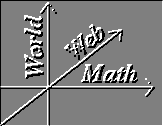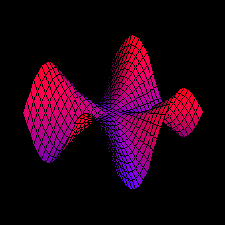#Vector Calculus IndexPaths:

Topics:
• Notation
• Summary
• Three-Dimensional Geometry
• N-tuples
• Cartesian Coordinates
• Vectors
• Vector Arithmetic
• Dot Product
• Matrices and Determinants
• Cross Product
• Parametric versus Implicit Descriptions
• Lines
• Planes
• Cylindrical Coordinates
• Spherical Coordinates
• N Dimensional Geometry
• Curves
• Velocity, Speed, and Acceleration
• Reparameterization
• Arc length
• Path integrals
• Work integrals
• Scalar valued functions
• Min/max
• Vector Fields
• Double and Triple Integrals
• Surfaces
• Fundamental Theorems of Vector Calculus

World Web Math Main Directory

watko@mit.edu